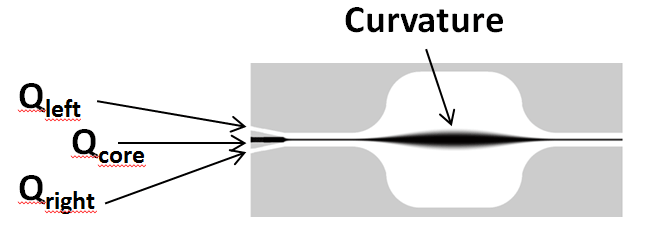Optofluidics refers to the technology that combines optics and microfluidics. It has applications in biosensors, displays, lab-on-chip devices, molecular imaging and lenses. These applications use optofluidic systems either to illuminate micro-scale bodies or to trigger stimuli to light in cellular bodies. In this blog, I will describe a FLOW-3D validation case study on dynamically configurable lenses, specifically L2 lenses. The validation references research conducted by Tang et al. [ref] for simulation setup and collecting experimental data.

## L2 Lenses

L2 lenses use the principle of light refraction. As light travels between media of different refractive indices, its speed changes and it bends. The formation of the lens itself however, is based on a simple microchannel design using the principles of fluid dynamics. This schematic shows the outline of the L2 lens (curvature) formed inside the expansion chamber because of the flow rates of the cladding and core inlets. Based on the curvature of the lens, light can be focused at focal points inside the beam-tracing chamber.(a) Schematic of the experimental setup for focusing light exiting an optical fiber through the L2 lens. (b) Field image of the L2 lens in operation

L2 (liquid-core liquid-cladding) lenses have some advantages over traditional lenses. They are dynamically reconfigurable, which means that the shape of the lens (curvature and focal length) can be changed in real time. Since laminar flows are characteristic of microfluidic systems, the interface of the lens is very smooth despite any roughness in the encasing wall channels. Lastly, the integration and pre-alignment of the lenses is simple because they are co-fabricated with the microfluidic device.

## Curvature Estimation

Estimating curvatures is central to this case study. Therefore, a rigorous method was devised combining mathematical properties of parabolas and length measurements in FlowSight (FLOW-3D’s advanced post-processer).

The curvature of a curve is given by,

$latex \displaystyle {{\kappa }_{{curve}}}=\frac{{\frac{{{{d}^{2}}y}}{{d{{x}^{2}}}}}}{{{{{\left[ {1+{{{\left( {\frac{{dy}}{{dx}}} \right)}}^{2}}} \right]}}^{{3/2}}}}}$

In this case y is the equation of the parabola, which is given by,

$latex y=a{{(x-h)}^{2}}+k$

Therefore, the curvature of the parabola is given by,

$latex \displaystyle {{\kappa }_{{parabola}}}=\frac{{2a}}{{{{{\left[ {1+\left\{ {2a\left( {x-h} \right)} \right\}2} \right]}}^{{3/2}}}}}$

After some more cleaning up, the curvature equation is reduced to,

$latex \displaystyle {{\kappa }_{{x=0}}}=2\frac{{{{y}_{1}}-k}}{{{{{10}}^{{-8}}}}}$

At this point FlowSight can be used to measure k and y1.

## Validations

The case study involved finding the lens curvatures for different combinations of flow rates. However, the combined flow rates from core and cladding inlets were fixed to 10 mL/hr for fair comparison between all combinations. The case study was divided into two parts – variable core flow rate (VCF) and fixed core flow rate (FCF).### Variable Core Flow (VCF) Rate

Fixing the left and the right cladding inlet flow rates to the same value. In this case core flow inlet rate is allowed to be changed; hence this is the case with variable core flow rates. The animation below shows variable lens profile formation for different core inlet flow rates. Since the left and the right cladding inlet flow rates are fixed to the same value, only bi-convex (symmetrical) lenses are formed.

### Fixed Core Flow (FCF) Rate

Fixing the core inlet flow rate to 3 mL/hr. This is the case with a fixed core flow rate. The animation below shows the lens profiles for different cladding flow rates and a fixed core flow rate. In this case different lens types are formed, such as bi-convex, plano-convex and meniscus.

## Validation Results

Around 30 simulations were run in FLOW-3D for VCF and FCF combined, each corresponding to an experimental combination of core and cladding inlet flow rates. Here are the results for VCF and FCF.

Visually, the FLOW-3D and experimental results are in excellent agreement. The curvatures start flattening out once the lens becomes too fat, and the edges start approaching the walls. This is evident from the curvature values corresponding to the core flow rates greater than 8 mL/hr inVCF. Similarly, in FCF, the left and the right curvatures start flattening out corresponding to left cladding flow rate values less than 1.5 mL/hr and greater than 5.5 mL/hr respectively. Outside these range of values, curvatures increase with increased core flow rates and left cladding flow rates.

To get a quantitative comparison between the numerical and experimental results, regression analysis was performed for the curvature estimation for VCF, and, left and right curvature estimations for FCF. The R2 value was used to quantify the goodness of the validation. Here are the results of the analysis.Quantitatively, the values of R2 show excellent (close to 1.0) correlation between the experimental and FLOW-3D results. This further demonstrates FLOW-3D’s capability to very accurately capture the hydrodynamics of the L2 lens formation.

We consistently explore such new technologies as the L2 lens so that we can provide researchers with a simulation tool that helps them perform more design combinations and parametric studies with robust and accurate results.

### Reference

Tang S. K. Y., Stan C. A., Whitesides G. M., Dynamically reconfigurable liquid-core liquid-cladding lens in a microfluidic channel, Lab on a Chip, 2008.Subscribe to the Blog
Privacy *